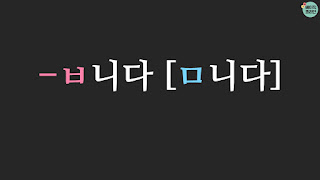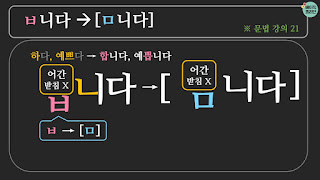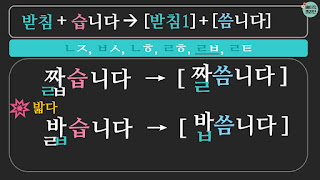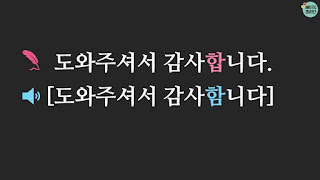기본 콘텐츠로 건너뛰기

### Learn Korean | Learn Korean Pronunciation: ㅂ니다 / 습니다

Welcome to Basick Korean!
In this lesson, we're going to learn how to pronounce 'ㅂ니다/습니다'

If you want to listen to this lesson in Korean (with English subtitles), please watch the below video.
🎬 Korean Pronunciation 'ㅂ니다/습니다': https://youtu.be/ELXBMWihB3Q

🌲Korean Pronunciation: ㅂ니다 / 습니다

(🌻 You can have this content in Korean.)

Let's look at how to pronounce 'ㅂ니다' first.the pronunciation of ㅂ니다

### 1. ㅂ니다 → [ㅁ니다]how to pronounce ㅂ니다

• 'ㅂ니다' is pronounced as [ㅁ니다]
• Grammar information
• an adjective/verb stem without final consonant + ㅂ니다
: 'ㅂ니다' attaches an adjective/verb stem without a final consonant, such as '하다 (to do) and 예쁘다 (to be pretty).' They become '합니다' and '예쁩니다.'
• 'ㅂ' in 'ㅂ니다' is placed at the final consonant position.
• Pronunciation
• When the final consonant 'ㅂ' is followed by 'ㄴ', 'ㅂ' is pronounced as [ㅁ].
• So 'ㅂ니다' is pronounced as [ㅁ니다].

Let's see some more examples.

• 합니다 is pronounced as [함니다]
• 하다 (to do) + ㅂ니다
• The final consonant 'ㅂ' is pronounced as [ㅁ]
• 예쁩니다 is pronounced as [예쁨니다]
• 예쁘다 (to be pretty) + ㅂ니다
• The final consonant 'ㅂ' is pronounced as [ㅁ].the final consonant 'ㄹ' and 'ㅂ니다' pronunciation

• In the case of 'ㄹ' final consonant
• When an adjective/verb stem ends with the final consonant ‘ㄹ', delete ‘ㄹ’ and attach ‘ㅂ니다.
• So in this case the final consonant 'ㄹ' is not pronounced.
• 만듭니다 is pronounced as [만듬니다].
• 만들다 (to make)(delete 'ㄹ') + ㅂ니다
• The final consonant 'ㅂ' is pronounced as [ㅁ].
• 놉니다 is pronounced as [놈니다].
• 놀다 (to play)(delete 'ㄹ') + ㅂ니다
• The final consonant 'ㅂ' is pronounced as [ㅁ].

Now, let's find out how to pronounce '습니다.'the pronunciation of 습니다

### 2. 습니다 → [씀니다]

#### 2.1 홑받침 (Single Final Consonants):      the final consonant+ 습니다 ⟶ [the final consonant sound] + [씀니다]how to pronounce '습니다'

• '습니다' is pronounced as [씀니다]
• Grammar information
• an adjective/verb stem with final consonant + 습니다
: '습니다' attaches an adjective/verb stem with a final consonant, such as '먹다 (to eat) and '덥다 (to be warm/hot).' They become '먹습니다' and '덥습니다.'
• Pronunciation
• '습니다' is pronounced as [슴니다].
• When the final consonant 'ㅂ' is followed by the initial consonant 'ㄴ', 'ㅂ' is pronounced as [ㅁ]. So '습니다' is pronounced [슴니다].
• [받침소리] + [씀니다]
• And before [슴니다], there is a final consonant from an adjective/verb stem.
• When they meet together, the final consonant is pronounced as [the final consonant sound] and [슴니다] is pronounced as [씀니다].
• The exception is 'ㅎ'.
•  The final consonant 'ㅎ' is not pronounced. Only  [씀니다] is pronounced.
Let's look at them one by one.

#### 2.1.1. [ㄱ]: ㄱ, ㄲㄱ, ㄲ + 습니다 ➝ [ㄱ] + [씀니다]

• ㄱ, ㄲ + 습니다 ➝ [ㄱ] + [씀니다]
• The final consonants 'ㄱ and ㄲ' are pronounced as the final consonant sound [ㄱ].
• 먹습니다 is pronounced as [먹씀니다].
• 먹다 (to eat) + 습니다
• The final consonant  'ㄱ' is pronounced the same.
• '습' is pronounced as [씀].
• 낚습니다 is pronounced as [낙씀니다].
• 낚다 (to fish, to catch) + 습니다
• The final consonant 'ㄲ' is pronounced as [ㄱ].
• 습' is pronounced as [씀].

#### 2.1.2. [ㄴ]: ㄴ

• ㄴ + 습니다 ➝ [ㄴ] + [씀니다]
• The final consonants 'ㄴ' is pronounced the same [ㄴ].
• 신습니다 is pronounced as [신씀니다].
• 신다 (to wear) + 습니다
• The final consonant  'ㄴ' is pronounced the same.
• '습' is pronounced as [씀].

#### 2.1.3. [ㄷ]: ㄷ, ㅅ, ㅆ, ㅈ, ㅊ, ㅌ

• ㄷ, ㅅ, ㅆ, ㅈ, ㅊ, ㅌ + 습니다 ➝ [ㄷ] + [씀니다]
• The final consonants 'ㄷ, ㅅ, ㅆ, ㅈ, ㅊ, ㅌ' is pronounced as the final consonant sound [ㄷ].
• 닫습니다 is pronounced as [닫씀니다].
• 닫다 (to close) + 습니다
• The final consonant  'ㄷ' is pronounced the same.
• '습' is pronounced as [씀].
• 같습니다 is pronounced as [갇씀니다].
• 같다 (to be same) + 습니다
• The final consonant 'ㅌ' is pronounced as [ㄷ].
• 습' is pronounced as [씀].

#### 2.1.4. [ㅁ]: ㅁ

• ㅁ + 습니다 ➝ [ㅁ] + [씀니다]
• The final consonants 'ㅁ' is pronounced the same [ㅁ].
• 검습니다 is pronounced as [검씀니다].
• 검다 (to be black) + 습니다
• The final consonant  'ㅁ' is pronounced the same.
• '습' is pronounced as [씀].

#### 2.1.5. [ㅂ]: ㅂ, ㅍ

• ㅂ, ㅍ + 습니다 ➝ [ㅂ] + [씀니다]
• The final consonants 'ㅂ, ㅍ' is pronounced as the final consonant sound [ㅂ].
• 덥습니다 is pronounced as [덥씀니다].
• 덥다 (to be warm/hot) + 습니다
• The final consonant  'ㅂ' is pronounced the same.
• '습' is pronounced as [씀].
• 깊습니다 is pronounced as [깁씀니다].
• 깊다 (to be deep) + 습니다
• The final consonant 'ㅍ' is pronounced as [ㅂ].
• 습' is pronounced as [씀].

####ㅎ + 습니다 ➝ ∅ + [씀니다]

• ㅎ + 습니다 ➝ ∅ + [씀니다]
• The final consonants 'ㅎ' is not pronounced.
• 좋습니다 is pronounced as [조씀니다].
• 좋다 (to be good) + 습니다
• The final consonant 'ㅎ' is not pronounced.
• '습' is pronounced as [씀].
• 낳습니다 is pronounced as [조씀니다].
• 낳다 (to give birth) + 습니다
• The final consonant 'ㅎ' is not pronounced.
• '습' is pronounced as [씀].

#### 2.2. 겹받침 (Double Final Consonants)Double Final Consonants + 습니다
• There are 11 double final consonants in Korean. But, 'ㄳ, ㄽ'  are not in adjectives and verbs. So we're going to look at 9 double final consonants.
• 1) 'ㄵ, ㅄ, ㄶ, ㅀ, ㄼ, ㄾ': the first final consonant is pronounced before '습니다.'
• 2) 'ㄺ, ㄻ, ㄿ': the second consonant is pronounced before '습니다.'
Let's look at them one by one.

#### 2.1.1. 'ㄵ, ㅄ, ㄶ, ㅀ, ㄼ, ㄾ' + 습니다 ➝ the first consonant + [씀니다]

• ㄵ + 습니다 ➝ [ㄴ] + [씀니다]
• 앉습니다 is pronounced as [안씀니다].
• 앉다 (to sit) + 습니다
• The first consonant 'ㄴ' is pronounced.
• So '앉' is pronounced as [안].
• '습' is pronounced as [씀].

• ㅄ + 습니다 ➝ [ㅂ] + [씀니다]
• 없습니다 is pronounced as [업씀니다].
• 없다 (not to exit) + 습니다
• The first consonant 'ㅂ' is pronounced.
• So' 없' is pronounced as [업].
• '습' is pronounced as [씀].ㄶ,ㅀ + 습니다 ➝ the first final consonant [ㄴ, ㄹ] + [씀니다]
• ㄶ + 습니다 ➝ [ㄴ] + [씀니다]
• 많습니다 is pronounced as [만씀니다].
• 많다 (to be many/much) + 습니다
• The first consonant 'ㄴ' is pronounced.
• So '많' is pronounced as [만].
• '습' is pronounced as [씀].

• ㅀ + 습니다 ➝ [ㄹ] + [씀니다]
• 앓습니다 is pronounced as [알씀니다].
• 앓다 (to be sick) + 습니다
• The first consonant 'ㄹ' is pronounced.
• So' 앓' is pronounced as [알].
• '습' is pronounced as [씀].ㄼ + 습니다 ➝ the first final consonant [ㄹ] + [씀니다], exception '밟다'

• ㄼ + 습니다 ➝ [ㄹ] + [씀니다]
• 짧습니다 is pronounced as [짤씀니다].
• 짧다 (to be short) + 습니다
• The first consonant 'ㄴ' is pronounced.
• So '짧' is pronounced as [짤].
• '습' is pronounced as [씀].

• 밟다 (to step on) is an exception.
• The second consonant 'ㅂ' is pronounced in the case of '밟다 '.
• 밟다+ 습니다 ➝ [ㅂ] + [씀니다]
• 밟습니다 is pronounced as [밥씀니다].
• 밟다 (to step on) + 습니다
• The second consonant 'ㅂ' is pronounced.
• So' 밟' is pronounced as [밥].
• '습' is pronounced as [씀].ㄾ + 습니다 ➝ the first final consonant [ㄹ] + [씀니다]

• ㄾ + 습니다 ➝ [ㄹ] + [씀니다]
• 핥습니다 is pronounced as [할씀니다].
• 핥다 (to lick) + 습니다
• The first consonant 'ㄴ' is pronounced.
• So '짧' is pronounced as [짤]
• '습' is pronounced as [씀].

#### 2.1.2. 'ㄺ, ㄻ, ㄿ' + 습니다 ➝ the second consonant + [씀니다]

• ㄺ + 습니다 ➝ [ㄱ] + [씀니다]
• 읽습니다 is pronounced as [익씀니다].
• 읽다 (to read) + 습니다
• The second consonant 'ㄴ' is pronounced.
• So '잃' is pronounced as [익].
• '습' is pronounced as [씀].

• ㄻ + 습니다 ➝ [ㅁ] + [씀니다]
• 굶습니다 is pronounced as [익씀니다].
• 굶다 (to starve, to skip a meal) + 습니다
• The second consonant 'ㅁ' is pronounced.
• So '굶' is pronounced as [굼].
• '습' is pronounced as [씀].ㄿ + 습니다 ➝ the second final consonant [ㅂ] + [씀니다]

• ㄿ + 습니다 ➝ [ㅂ] + [씀니다]
• 읊습니다 is pronounced as [읍씀니다].
• 읊다 (to recite) + 습니다
• The second consonant 'ㅍ' is pronounced and 'ㅍ' is pronounced as the final consonant sound [ㅂ].
• So '읊' is pronounced as [읍].
• '습' is pronounced as [씀].

We've looked at all the final consonants.
Now let's see two sentences.

#### 3. Example sentences'ㅂ니다 [ㅁ니다]' example sentence

• When you write, you have to write '도와주셔서 감사합니다' which means 'Thank you for helping me out.'
• But when you pronounce, you have to pronounce [도와주셔서 감사함니다].
• '합' is pronounced as [함].'습니다 [씀니다]' example sentence

• When you write, you have to write '도와주셔서 고맙습니다' which means 'Thank you for helping me out.'
• But when you pronounce, you have to pronounce [도와주셔서 고맙씀니다].
• '습' is pronounced as [씀].

Today we've learned how to pronounce 'ㅂ니다/습니다.'

Here are the related videos on Youtube.
More videos on Korean pronunciation: http://bit.ly/basickoreanpronunciation

Thank you~!

### Learn Korean: Korean Grammar 125: V-아다 주다/어다 주다/해다 주다 (vs 아 주다/어 주다/해 주다)

In this lesson, we’re going to learn ‘ V-아다 주다/어다 주다/해다 주다 ’ which  is used  to ask someone to do something or  you are doing something for the sake of others. ‘편의점에서 커피 좀 사다 주세요.’ (Can you buy me some coffee at the convenience store?)

### Learn Korean Grammar 81: A/V으니까/니까, N이니까/니까

Hello~Welcome to Basic Korean. In this lesson, we're going to learn a Korean grammar 'A/V으니까/니까, N이니까/니까' If you want to listen to this lesson in Korean (with English subtitles), please watch the video below.  🎬 Korean Grammar Video 81:  https://youtu.be/xmgheSDPtaI 🌲 Korean Grammar 81: A/V -으느까/니까, N -이니까/니까 (You can have this post in Korean. ) Let's look at examples first and see how today's grammar is used. 1. A short conversation - The  [sky blue]  is pronunciation. [Translation] - 미소: 우리 이번 주말에 어디로 놀러 갈까요?             (Where shall we go this weekend?)          - 나나: 날씨가 더우니까 바다로 갈까요?             (Because the weather is hot, shall we go to the beach?) - 미소: 좋아요.              (That sounds good.)        Here, today's grammar is '더우니까' Let's look at today's grammar! 2. Usage '(으)니까' attaches after an adjective/verb stem. '(이)니까' attaches after a noun. It is a connective ending and it is used to indicate that the preceding sta

### Learn Korean | Learn Korean Grammar 02: N이에요/예요

Hello~Welcome to Basic Korean. In this lesson, we're going to learn a Korean grammar 'N이에요/예요.' If you want to listen to this lesson in Korean (with English subtitles), please watch the below video.  🎬 Korean Grammar Video 02:  https://youtu.be/xwJADfXcxNA 🌲 Korean Grammar 02: N -이에요/예요 (You can have this content  in Korean. ) Let's look at examples first and see how today's grammar is used. 1. A short conversation Korean Grammar '이에요/예요' example [Translation] - 제 이름은 이광수예요. (My name is Lee Gwangsoo.) - 저는 한국 사람이에요. (I am Korean.) Here, today's grammar is '광수예요, 한국 사람이에요.' Let's look at today's grammar! 2. Usage Korean Grammar '이에요/예요' usage '이에요/예요' attaches after a noun. It shows the state or attribute of the subject/topic of the sentence. '이에요/예요' is similar to 'to be' verb in English. Let's look at two example sentences. Korean Grammar '이에요/예요' usage ✎  '제 이름은 이광수예요.' which means

### 한국어 배우기 | 한국어 문법 75: V-아서/어서/해서

안녕하세요~ 베이직 코리안입니다. 오늘은 수단이나 방법을 이야기할 때 사용하는 표현 'V-아서/어서/해서'를 알아봅시다! 🎬 한국어 문법 강의:  https://youtu.be/0caAw7IHPZQ 🌱 강의 노트 – 한국어 문법 75: 아서/어서/해서 (3) 방법, 수단 1. 예문 - 나나: 미소 씨, 요즘 다이어트해요? 살이 많이 빠졌어요. - 미소: 네, 요즘 운동해서 살을 빼고 있어요. 2. 용법 1) 연결어미 2) 동사와 결합 2) 앞에 오는 행동이 뒤에 오는 일의 수단이나 방법을 나타낼 때 사용 . 3) 예문 1) 저는 운동해서 살을 빼고 있어요. 2) 손을 자주 씻어서 코로나 19 감염을 예방해요. 3. 결합정보 1) 모음 ㅏ, ㅗ: 아서 - 가다 + 아서 → 가서 - 오다 + 아서 → 와서 2) 나머지 모음: 어서 - 입다 + 어서 → 입어서 - 만들다 + 어서 → 만들어서 3) 하다 → 해서 - 운동하다 → 운동해서 - 공부하다 → 공부해서 4. 주의 사항 1) 앞 문장 주어 = (뒤 문장 주어) - 나는 컴퓨터를 켜서 오빠는 영화를 봤어요. (X) (주어: 나 ≠ 오빠) - 나는 컴퓨터를 켜서 (나는) 영화를 봤어요. (O) (주어: 나 = 나) 2) 시제표현 X + 아서/어서/해서 - 저는 컴퓨터를 켰어서 영화를 봤어요. (켰어서 X) - 저는 컴퓨터를 켜서 영화를 봤어요. (켜서 O) 5. 아서/어서/해서 비교 (1) 시간 순서에 따라 두 행동을 연결 - 놀이동산에 가서 롤러코스터를 타요. - 동사와 결합 - 주어 일치 O - 시제표현 X - 모든 문장에서 사용 O (2) 이유/원인을 나타낼 때 - 아파서 병원에 가요 - 동사/형용사와 결합 - 주어 일치 O - 시제표현 X - 명령문, 청유문에서는 사용할 수 없음 (3) 수단/방법을 나타낼 때 - 운동해서 살을 빼요. - 동사와 결합 - 주어 일치 O - 시제표현 X - 모든 문장에서 사용 O 6. 예문 - 김치를 볶아서 먹

### Learn Korean | Learn Korean Grammar 76: 'A은/ㄴ 것 같다, V는 것 같다, N인 것 같다'

Hello~Welcome to Basic Korean. In this lesson, we're going to learn a Korean grammar 'A은/ㄴ 것 같다, V는 것 같다, N인 것 같다' If you want to listen to this lesson in Korean (with English subtitles), please watch the below video.  🎬 Korean Grammar Video 76:  https://youtu.be/h_qa75iyjsE   🌲 Korean Grammar 76: A 은/ㄴ 것 같다,  V 는 것 같다 ,  N 인 것 같다 🌻 You can have this content  in Korean. Let's look at a short conversation first and see how today's grammar is used. 1. A short conversation The [sky blue] is pronunciation. Grammar 76: a short conversation with pronunciation [Translation] - 지안: 나나 씨, 혹시 미소 씨 어디에 있는지 알아요?            (Nana, do you know where Miso is?)           전화를 안 받네요.            (She does not answer the phone.) - 나나: 미소 씨는 지금 회의실에 있는 것 같아요.           (It seems that Miso is in the meeting room.)           아까 회의실에 간다고 했어요.           (She said she went to the meeting room.) Here, today's grammar is '있는 것 같아요.' Let's look at today's grammar! 2. Usa

### 한국어 배우기 | 한국어 문법 80: ㅅ불규칙

안녕하세요. 베이직 코리안입니다. 오늘은 한국어 문법 'ㅅ불규칙'을 알아보겠습니다. 한국어로 이 강의를 들으시려면 아래 링크를 클릭해 주세요! 🎬 한국어 문법 강의 80:  https://youtu.be/_OxEeABKYG4   🌲 한국어 문법 80: ㅅ 불규칙 (You can have this post  in English. ) 먼저 오늘의 문법이 문장에서 어떻게 쓰이는지 예문 볼게요. 1. 예문 -  [발음] 입니다. ㅅ 불규칙 예문 [Translation] - 나나: 미소 씨, 감기는 다 나았어요 ?          - 미소: 아니요. 약을 먹었는데도 잘 낫지 않네요 . 여기에서 오늘 배울 문법은 '나았어요, 낫지 않네요.'입니다. 모두 동사 '낫다'에서 활용한 표현이에요. 그럼 오늘의 문법 자세히 알아볼게요. 2. ㅅ불규칙 2.1 낫지 않네요 vs 나았어요 ㅅ불규칙 '낫지 않네요'와 '나았어요'를 비교해 볼게요. 먼저 '낫지 않네요'를 볼게요. 낫지 않네요: 낫다 + 지 않네요 '낫지 않네요'는 동사 '낫다' 뒤에 자음으로 시작하는 어미 '지 않네요'가 경우에는 '낫지 않네요'라고 이야기해요. '낫'에 있는 받침 'ㅅ'이 그대로 유지돼요.  반대로 나았어요: 낫다 + 았어요 '나았어요'는 동사 '낫다' 뒤에 모음으로 시작하는 어미 '았어요'가 결합하는 경우에는 '낫았어요'라고 이야기하면 틀립니다. '낫'의 받침 'ㅅ' 뒤에 모음으로 시작하는 어미가 오는 경우에는, 받침 'ㅅ'을 지우고 뒤에 '았어요'를 사용해요. 그래서 '나았어요'라고 이야기합니다. 그리고 이런 현상을 'ㅅ불규칙'이라고 해요. 그럼 'ㅅ불규칙'을 조금 더

### Learn Korean | Korean Grammar 131: N-이라고 하다/라고 하다. (indirect quotation)

Welcome to Basic Korean. In this lesson, we will look at the expression 'N-이라고 하다/라고 하다' which is used to indirectly quote a sentence whose type of sentence is 'declarative sentence', ends with 'noun', and the point of time is 'the present tense'. Let's learn 'indirect quotation' in Korean 'N-이라고 하다/라고 하다.'